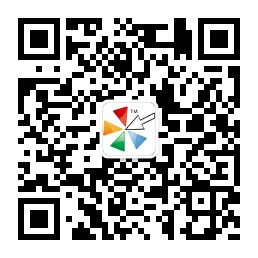0712-2888027 189-8648-0214### PHPCMS中PC:GET标签完全使用指南

{get dbsource=" " sql=" "}

{/get}

1.get标签属性值必须用双引号括起来( " " )。

{get sql=" " /}

2.get标签必须含有结束标记，即正确get标签必须是成对出现：整个Get标签含有结束标记“ {/get}”，或者是“/”。

3.get标签里面含有的变量，数组和函数必须用“{}”包括。

{str_cut(\$r[title], 50)}
{\$r[url]}

4.get标签 sql语句中条数限制。( rows=" " )

{get sql=" " rows="10"}
{/get}

5.get标签 sql语句中条件限制。( where )

{get sql="select * from phpcms_content where catid=1"}

{/get}

6.get标签 sql语句中排序。( order by )

{get sql="select * from phpcms_content order by updatetime desc" }
{\$r[title} URL：{\$r[url]}
{/get}

7.get标签里面嵌套php函数。(可以参考上面的第3点)

{date('Y-m-d', \$r[updatetime])}

8.本系统数据调用(当前数据库调用)

{get sql=""}
{/get}

{get sql="" return="r"}
{/get}

9.本系统数据调用10条

{get sql="" rows="10"}
{/get}

10.同一个数据库帐号的不同数据库调用

{get dbname="" sql="" }
{/get}

11.不同数据库帐号调用(不同数据源调用)

{get dbsource="" sql=""}
{/get}

12.不同数据库帐号的不同数据库调用

{get dbsource="" dbname="" sql="" }

{/get}

13.本系统数据调用，带分页

{get sql=" " page="\$page"}
{/get}

14.本系统数据调用，自定义返回变量

{get sql=" " return="v"}
{\$v[ ]}
{/get}

*get 标签参数完整剖析

{get dbsource="数据源" dbname="数据库" sql="SQL语句" rows="行数" return="返回变量名称" page="\$page"}

{/get}

dbname="数据库" -->
(数据库 在添加数据源的时候需要填写的数据库名)

sql="SQL语句" -->
SQL语句可以参考Mysql手册，上面get 标签语法第4、5、6点和SQL语句剖析已经提及要点

rows="行数" -->

return="返回变量名称" -->

page="\$page" -->

*SQL语句剖析

sql="select 字段 from 表名 where 条件表达式 order by 字段 desc/asc"

6条完整get 标签代码示例

1.调用本系统单条数据，示例（调用ID为1的信息，标题长度不超过25个汉字，显示更新日期）：

{get sql="select * from phpcms_content where contentid=1" /}

2.调用本系统多条数据，示例（调用栏目ID为1通过审核的10条信息，标题长度不超过25个汉字，显示更新日期）：

{get sql="select * from phpcms_content where catid=1 and status=99 order by updatetime desc" rows="10"}

{/get}

3.带分页，示例（调用栏目ID为1通过审核的10条信息，标题长度不超过25个汉字，显示更新日期，带分页）：

{get sql="select * from phpcms_content where catid=1 and status=99 order by updatetime desc" rows="10" page="\$page"}

{/get}

4.自定义返回变量，示例（调用栏目ID为1通过审核的10条信息，标题长度不超过25个汉字，显示更新日期，返回变量为 \$v）：

{get sql="select * from phpcms_content where catid=1 and status=99 order by updatetime desc" rows="10" return="v"}

{/get}

5.调用同一帐号下的其他数据库，示例（调用数据库为bbs，分类ID为1的10个最新主题，主题长度不超过25个汉字，显示更新日期）：

{get dbname="bbs" sql="select * from cdb_threads where fid=1 order by dateline desc" rows="10"}

{/get}

6.调用外部数据，示例（调用数据源为bbs，分类ID为1的10个最新主题，主题长度不超过25个汉字，显示更新日期）：

{get dbsource="bbs" sql="select * from cdb_threads where fid=1 order by dateline desc" rows="10"}

{/get}

1、获取get 标签代码

{get sql="SELECT `contentid`,`title`,`description`,`url`,`inputtime` FROM `phpcms_content` WHERE `contentid` = '1' ORDER BY `contentid` DESC"rows="10" page="\$page" }
{\$r[contentid]}
{\$r[title]}
{\$r[description]}
{\$r[url]}
{\$r[inputtime]}
{/get}
{\$pages}

{get sql="SELECT `contentid`,`title`,`description`,`url`,`inputtime` FROM ` phpcms_content`" WHERE `contentid` = '1' ORDER BY   `contentid` DESC   rows="10"   page="\$page" }
//条件是ID=1，排序按ID降序排序，显示10行，带分页

{\$r[contentid]}
// ID

{\$r[title]}
//标题

{\$r[description]}
//摘要

{\$r[url]}
//链接地址

{\$r[inputtime]}
//发布时间
{/get}
{\$pages}
//分页

2、将代码插入到模板文件

get 标签代码如下：
{get dbsource="discuz" sql="SELECT `author`,`subject`,`lastpost` FROM `cdb_threads` ORDER BY `lastpost` DESC" rows="3" }
{\$r[author]}
{\$r[subject]}
{\$r[lastpost]}
{/get}

{get dbsource="discuz" sql="SELECT `author`,`subject`,`lastpost` FROM `cdb_threads` ORDER BY `lastpost` DESC" rows="3" }
({\$r[author]}){str_cut(\$r[subject], 22,"")} date:{date("Y-m-d",\$r[lastpost])}

{/get}